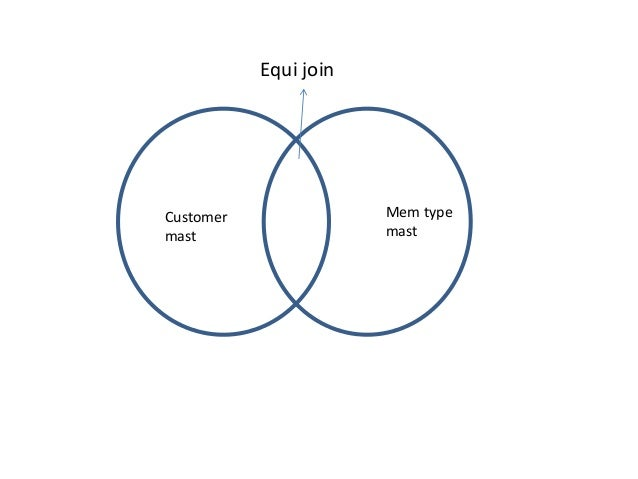Equi join Vs Inner Join top differences to read today

The equi JOINs and Inner JOINs are tricky in SQL. Many programmers think that both are same, but unable to tell where the functionally is different. So, I have selected this point to explain clearly where both differ each other.

Equi JOIN

The term equi join means joining two are more tables based on equal condition.In the above example there are two tables ‘Customer mast’ and ‘Mem type mast’. The arrow mark shows that result of equi joins.

Equi joins

• The Joins are formed based on equal ‘=’ condition in ‘WHERE’ clause . I mean to say that in ‘WHERE’ clause you can give ‘>’ or ‘<‘ condition. But in equi-join scenario only eaual condition is given.
• The result of equi join is the the matching rows will be joined based on the equal matching columns in both (or more) tables
• Do not confuse that a join can be formed on two or more tables.
• In equi-join the join condition is usually given in ‘WHERE’ clause

Example equi Join

The first table is “Customermast”The second Table is “Memtypemast”SELECT DEPT, PROJ
FROM CUSTOMERMAST C, MEMTYPEMAST M
WHERE C.ROLE = M.ROLE;

The result of equi Join is as follows

The term inner join means only matching rows in the first table are included. There is no concept of ‘LEFT INNER JOIN’ or ‘RIGHT INNER JOIN’Inner Join

• In inner join, only matching rows of first table (TABLE A),  will be formed in the result
• SQL query first it looks for each matching row of TABLE-A in TABLE-B. If matching rows present, those rows formed into result table
• You can give in inner join any condition like ‘=’,'<‘ and ‘>’. Here, it differentiates with equi Join.
• The above SQL query is also applicable for Inner join.